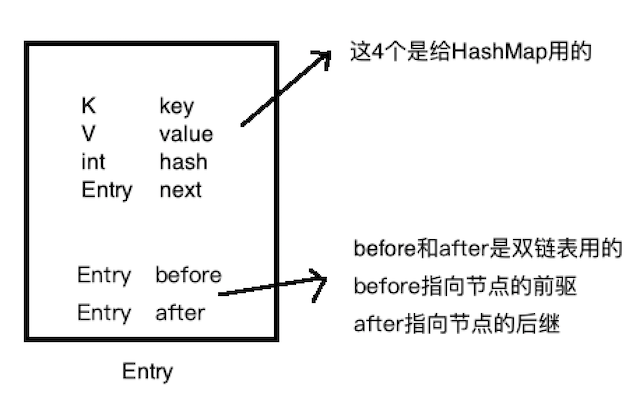## 概述

LruCache的缓存算法

LruCache采用的缓存算法为LRU（Least Recently Used），最近最少使用算法。核心思想是当缓存满时，会首先把那些近期最少使用的缓存对象淘汰掉

LruCache的实现

LruCache底层就是用LinkedHashMap来实现的。提供 get 和 put 方法来完成对象的添加和获取

相同点：
1. 都是key,value进行添加和获取
2. 底层都是使用数组来存放数据

2. LinkedHashMap内存的节点存放在数据中，但是节点内部有两个指针，来完成双向链表的操作，来保证节点插入顺序或者访问顺序

    public static void main(String[] args) {

//默认记录的就是插入顺序
Map<String, String> map = new LinkedHashMap<>();
map.put("name", "tom");
map.put("age", "34");

Iterator iterator = map.entrySet().iterator();

//遍历
while (iterator.hasNext()) {
Map.Entry entry = (Map.Entry) iterator.next();
String key = (String) entry.getKey();
String value = (String) entry.getValue();
System.out.println("Key = " + key + ", Value = " + value);
}
}

Key = name, Value = tom
Key = age, Value = 34
Key = address, Value = beijing

HashMap的遍历

  public static void main(String[] args) {

//插入和上面一样的值
Map<String, String> map = new HashMap<>();
map.put("name", "tom");
map.put("age", "34");

Iterator iterator = map.entrySet().iterator();

//遍历
while (iterator.hasNext()) {
Map.Entry entry = (Map.Entry) iterator.next();
String key = (String) entry.getKey();
String value = (String) entry.getValue();
System.out.println("Key = " + key + ", Value = " + value);
}
}

Key = address, Value = beijing
Key = name, Value = tom
Key = age, Value = 34

1. HashMap遍历的时候，是无序的，和插入的顺序是不相关的。

    public static void main(String[] args) {

/**
* 第一个参数：数组的大小
* 第二个参数：扩容因子，和HashMap一样，添加的元素的个数达到 16 * 0.75时，开始扩容
* 第三个参数：true:表示记录访问顺序
*           false:表示记录插入顺序（默认的顺序）
*/
Map<String, String> map = new LinkedHashMap<>(16,0.75f,true);

//分别插入下面几个值
map.put("name", "tom");
map.put("age", "34");

//既然演示访问顺序，我们就访问其中一个元素，这里只是打印一下
System.out.println("我是被访问的元素：" + map.get("age"));

//访问完后，我们再遍历，注意输出的顺序
Iterator iterator = map.entrySet().iterator();
//遍历
while (iterator.hasNext()) {
Map.Entry entry = (Map.Entry) iterator.next();
String key = (String) entry.getKey();
String value = (String) entry.getValue();
System.out.println("Key = " + key + ", Value = " + value);
}
}

我是被访问的元素：34
Key = name, Value = tom
Key = address, Value = beijing
Key = age, Value = 34

LinkedHashMap中的节点如下图static class QEntry<K, V> {
public K key;      //key
public V value;    //value
public int hash;   //key对应的hash值
public QEntry<K, V> next;   //hash冲突时，构成一个单链表

public QEntry<K, V> before; //当前节点的前一个节点
public QEntry<K, V> after;  //当前节点的后一个节点

QEntry(K key, V value, int hash, QEntry<K, V> next) {
this.key = key;
this.value = value;
this.hash = hash;
this.next = next;
}

//删除当前节点
private void remove() {
//当前节点的上一个节点的after指向当前节点的下一个节点
this.before.after = after;
//当前节点的下一个节点的before指向当前节点的上一个节点
this.after.before = before;
}

//将当前节点插入到existingEntry节点之前
private void addBefore(QEntry<K, V> existingEntry) {

//插入到existingEntry前，那么当前节点后一个节点指向existingEntry
this.after = existingEntry;

//当前节点的上一个节点也需要指向existingEntry节点的上一个节点
this.before = existingEntry.before;

//当前节点的下一个节点的before也得指向自己
this.after.before = this;

//当前节点的上一个节点的after也得指向自己
this.before.after = this;
}

//访问了当前节点时，会调用这个函数
//在这里面就会处理访问顺序和插入顺序

//如果accessOrder为true，也就是访问顺序
if (lm.accessOrder) {

//把当前节点从链表中删除
remove();

//再把当前节点插入到双向链表的尾部
}
}
}

public class QLinkedHashMap<K, V> {
private static int DEFAULT_INITIAL_CAPACITY = 16;   //默认数组的大小
private static float DEFAULT_LOAD_FACTOR = 0.75f;   //默认的扩容因子

private QEntry[] table;     //底层的数组
private int size;           //数量

//下面这两个属性是给链表用的

//true:表示按着访问的顺序保存   false:按照插入的顺序保存（默认的方式）
private boolean accessOrder;

//双向循环链表的表头,记住，这里只有一个头指针，没有尾指针
//所以需要用循环链表来实现双向链表
//即：从前可以往后遍历，也可以从后往前遍历

//创建DEFAULT_INITIAL_CAPACITY大小的数组
table = new QEntry[DEFAULT_INITIAL_CAPACITY];
size = 0;

//默认按照插入的顺序保存
accessOrder = false;

//初始化
init();
}

/**
*
* @param capcacity     数组的大小
* @param accessOrder   按照何种顺序保存
*/
public QLinkedHashMap(int capcacity, boolean accessOrder) {
table = new QEntry[capcacity];
size = 0;

this.accessOrder = accessOrder;
init();
}

//这里主要是初始化双向循环链表
private void init() {
//新建一个表头
header = new QEntry<>(null, null, -1, null);

//链表为空的时候，只有一个头节点，所以头节点的下一个指向自己，上一个节点也指向自己
}

//插入一个键值对
public V put(K key, V value) {
if (key == null)
throw new IllegalArgumentException("key is null");

//拿到key的hash值
int hash = hash(key.hashCode());
//存在数组中的哪个位置
int i = indexFor(hash, table.length);

//看看有没有key是一样的，如果有，替换掉旧掉，把新值保存起来
//如调用了两次 map.put("name","tom");
// map.put("name","jim"); ，那么最新的name对应的value就是jim
QEntry<K, V> e = table[i];
while (e != null) {
//查看有没有相同的key,如果有就保存新值，返回旧值
if (e.hash == hash && (key == e.key || key.equals(e.key))) {
V oldValue = e.value;
e.value = value;

//重点就是这一句，找到了相同的节点,也就是访问了一次
//如果accessOrder是true，就要把这个节点放到链表的尾部
e.recordAccess(this);

//返回旧值
return oldValue;
}

//继续下一个循环
e = e.next;
}

//如果没有找到与key相同的键
//新建一个节点，放到当前 i 位置的节点的前面
QEntry<K, V> next = table[i];
QEntry newEntry = new QEntry(key, value, hash, next);

//保存新的节点到 i 的位置
table[i] = newEntry;

//把新节点添加到双向循环链表的头节点的前面，
//因为这是一个双向循环链表，头节点的before指向链表的最后一个节点
//刚开始我也以为是添加到了链表的头部，其实不是，是添加到了链表的尾部
//这点可以参考图好好想想

//别忘了++
size++;

return null;
}

//根据key获取value，也就是对节点进行访问
public V get(K key) {

//同样为了简单，key不支持null
if (key == null) {
throw new IllegalArgumentException("key is null");
}

//对key进行求hash值
int hash = hash(key.hashCode());

//用hash值进行映射，得到应该去数组的哪个位置上取数据
int index = indexFor(hash, table.length);

//把index位置的元素保存下来进行遍历
//因为e是一个链表，我们要对链表进行遍历
//找到和key相等的那个QEntry，并返回value
QEntry<K, V> e = table[index];
while (e != null) {

//看看数组中是否有相同的key
if (hash == e.hash && (key == e.key || key.equals(e.key))) {

//访问到了节点，这句很重要，如果有相同的key，就调用recordAccess()
e.recordAccess(this);

//返回目标节点的值
return e.value;
}

//继续下一个循环
e = e.next;
}

//没有找到
return null;
}

//返回一个迭代器类，遍历用
public QIterator iterator(){
}

//根据 h 求key落在数组的哪个位置
static int indexFor(int h, int length) {
//或者  return h & (length-1) 性能更好
//这里我们用最容易理解的方式，对length取余数，范围就是[0,length - 1]
//正好是table数组的所有的索引的范围

h = h > 0 ? h : -h; //防止负数

return h % length;
}

//对hashCode进行运算，JDK中HashMap的实现，直接拷贝过来了
static int hash(int h) {
h ^= (h >>> 20) ^ (h >>> 12);
return h ^ (h >>> 7) ^ (h >>> 4);
}

//定义一个迭代器类，方便遍历用
public class QIterator {
QEntry<K,V> p;

}

//是否还有下一个节点
public boolean hasNext() {
}

//如果有下一个节点，获取之
public QEntry next() {
QEntry r = p;
p = p.after;
return r;
}
}

static class QEntry<K, V> {
public K key;      //key
public V value;    //value
public int hash;   //key对应的hash值
public QEntry<K, V> next;   //hash冲突时，构成一个单链表

public QEntry<K, V> before; //当前节点的前一个节点
public QEntry<K, V> after;  //当前节点的后一个节点

QEntry(K key, V value, int hash, QEntry<K, V> next) {
this.key = key;
this.value = value;
this.hash = hash;
this.next = next;
}

//删除当前节点
private void remove() {
//当前节点的上一个节点的after指向当前节点的下一个节点
this.before.after = after;
//当前节点的下一个节点的before指向当前节点的上一个节点
this.after.before = before;
}

//将当前节点插入到existingEntry节点之前
private void addBefore(QEntry<K, V> existingEntry) {

//插入到existingEntry前，那么当前节点后一个节点指向existingEntry
this.after = existingEntry;

//当前节点的上一个节点也需要指向existingEntry节点的上一个节点
this.before = existingEntry.before;

//当前节点的下一个节点的before也得指向自己
this.after.before = this;

//当前节点的上一个节点的after也得指向自己
this.before.after = this;
}

//访问了当前节点时，会调用这个函数
//在这里面就会处理访问顺序和插入顺序

//如果accessOrder为true，也就是访问顺序
if (lm.accessOrder) {

//把当前节点从链表中删除
remove();

//再把当前节点插入到双向链表的尾部
}
}
}
}

 public static void main(String[] args){
//新建一个默认的构造函数，默认是按照插入顺序保存
map.put("name","tom");
map.put("age","32");

//验证是不是按照插入的顺序打印
while (iterator.hasNext()){
QEntry e = iterator.next();
System.out.println("key=" + e.key + " value=" + e.value);
}
}

key=name value=tom
key=age value=32
key=address value=beijing

  public static void main(String[] args){
//新建一个大小为16，顺序是访问顺序的 map

//分别插入以下键值对
map.put("name","tom");
map.put("age","32");

//访问其中一个元素，这里什么也不做
//访问了age,那么打印的时候，age应该是最后一个打印的
map.get("age");

//验证是不是按照访问顺序打印，age是不是最后一个打印
while (iterator.hasNext()){
QEntry e = iterator.next();
System.out.println("key=" + e.key + " value=" + e.value);
}
}

key=name value=tom
key=age value=32